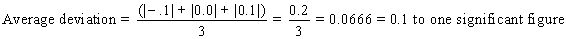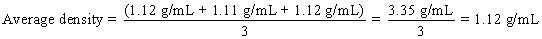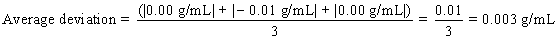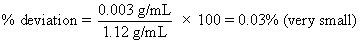# CHEM.1230L: Chemistry I Lab

## Overview

This experiment consists of three parts:

• measuring the density of an irregularly shaped solid,
• measuring the density of a unknown liquid sample
• making multiple measurements of the density of water.

This experiment also gives you practice in working with significant figures in measurements. In order to determine the density of a material, you need to determine the mass and the volume of a sample of the material. In the laboratory, it is far easier to determine the mass of an object precisely (using a balance) than it is to determine the volume of the object. This leads to some careful consideration of significant figures when calculating the density of the object (mass divided by volume).

Calculations:

Since you make several determinations of the density of each sample, it is possible to calculate a mean value for each of the densities, average deviation, and percent deviation. For a review of the calculation of average and percent deviations, see the Help file for Experiment 1 (or click here to go to that file).

Report Page 13

For Part C (Density of distilled water) note that the mass and volume readings should be cumulative after each additional amount of water is withdrawn from the buret and weighed. For example, line 3b should represent the total mass and the total volume after the second sample is withdrawn from the buret (not just the increased mass and increased volume since the first sample was withdrawn). Since we know the density of water is around 1 g/mL, we should see a roughly linear relationship between the masses and the volumes.

Density of Solid: Page 13, Part II A

Suppose the following data were recorded for the solid sample (see Page 13 Part A):

 Trial 1 Trial 2 Trial 3 Mass empty beaker 7.32 g 7.44 g 7.21 g Mass beaker + solid 18.52 g 18.55 g 18.46 g Volume water in cylinder 25.2 mL 24.1 mL 27.3 mL Volume water + solid 30.7 mL 29.2 mL 32.7 mL

#### 1. Volume of solid sample - calculation Trial 1

The solid caused the water level for Trial 1 to rise from 25.2 to 30.7 mL. Since the solid did not react with or dissolve in the water, the volume of the solid in mL is represented by just the change in water levels. Calculate the volume of the solid, then click here to check your answer.

#### 2. Mass of solid sample - calculation Trial 1

The mass of the solid sample is just the difference between the mass of the empty beaker and the mass of the beaker with the solid. Based on the data in the table above, calculate the mass of the solid sample for Trial 1, and then click here to check your answer.

#### 3. Density of Solid Sample - calculation Trial 1

The density of a sample represents the mass of the sample divided by the volume of the sample. For the solid sample in Trial 1, calculate the density, then click here to check your answer. Pay close attention to significant figures!

#### 4. Average Density of Solid Sample

Remember that you have to average the densities determined in all three trials (not just two).

For the data in the table above, the three individual densities as calculated from the data in the table (in the same manner as illustrated above for Trial 1) are as follows

 Trial 1 Trial 2 Trial 3 Density 2.0 g/mL 2.2 g/mL 2.1 g/mL

The average (mean) density is then the sum of the three densities divided by the number of trials (three). Calculate the average density, then click here to check your answer.

#### 5. Deviations in Density

Remember to calculate the deviations for all three trials.

For a review of deviation calculations, see Experiment 1 (or click here).

Deviation

Trial 1

(2.0 - 2.1) = -0.1 g/mL

Trial 2

(2.1 - 2.1) = 0.0 g/mL

Trial 3

(2.2 - 2.1) = 0.1 g/mL

#### 6. Average deviation in density of solid

Remember to calculate the average based on all three trials.

For a review of average deviation calculations, see Experiment 1 (or click here).Density of Liquid Unknown: Page 15, Section IIB

Remember that all calculations should be for all three trials (not just Trials 1 and 2).

Suppose the following data were recorded for the density of the liquid unknown (see Page 14, Part IB):

 Trial 1 Trial 2 Trial 3 Mass beaker + liquid 31.42 g 33.69 g 34.54 g Volume of liquid 21.6 mL 23.6 mL 23.7 mL Mass empty beaker 7.25 g 7.49 g 7.92 g

1. Volume of Liquid Sample for Trial 1

There is no calculation involved here. You measured the volume directly with the graduated cylinder. The volume for Trial 1 (see table above) is 21.6 mL.

2. Mass of Liquid Sample for Trial 1

This is just the difference in mass between the empty beaker and the beaker with the liquid in it! For Trial 1 in the table above, calculate the mass of the liquid sample, then click here to check your result.

3. Density of Liquid Sample for Trial 1

The density of a liquid sample is the mass of the sample divided by its volume. Since the mass is known to 4 significant figures, but the volume is only known to 3 significant figures, the calculated density should only be expressed to 3 significant figures. For the mass and volume for Trial 1, calculate the density of the liquid sample, then click here to check your answer.

4. Average Density of Liquid Sample

Remember that all three trials should be used (not just Trials 1 and 2).

The densities for the three trials (calculated as shown for Trial 1 above) and the average density are given below:

 Trial 1 Trial 2 Trial 3 Density 1.12 g/mL 1.11 g/mL 1.12 g/mL## Deviations in Density

5. Deviations in Density

Remember to calculate the deviations for all three trials.

 Trial 1 Trial 2 Trial 3 Deviation 0.00 g/mL -0.01 g/mL 0.00 g/mL

6. Average Deviation in Density of Liquid

Again, be sure to use all three trials, and remember that in calculating the average deviation it is the absolute value of the deviations which should be used.Note that because our three densities really agreed with each other well, we come up with an average deviation of effectively 0.00 g/mL (when significant figures are considered).

7. Percent Deviation in Density of Liquid## Density of Distilled Water: Page 15, Part 11C

1. Corrected Mass and Volume After Each Addition of Water

We don't really care what the beaker you used weighs, and we don't care to what level you filled the buret before you started taking water samples from it: we just need the mass of each water sample and its volume. Remember that we for each set of data you recorded in Part C on Page 13, we want the total cumulative mass and volume after each addition of water (not just the incremental amount since the previous edition. To calculate the mass after each addition, subtract the mass of the empty beaker from the current mass (with current amount of water present). To calculate the volume after each addition, subtract the initial reading of the buret before any water was dispensed from the current buret reading for a particular sample.

2. Graph

It is essential that you follow the graphing instructions given in the Appendix at the back of the lab manual for making your graph. Graphs count a lot in lab and you will receive major penalties if you do not follow the instructions provided. There is also a sample graph for this experiment posted in the laboratory.